FundooTutor

# EXERCISE 2.2

1. Find the zeros of the quadratic polynomials and verify the relationships between the zeros and the coefficients.

(i) x2-2x-8

Let, P(x) = x2-2x-8

Comparing P(x) with a quadratic equation ax2+bx+c

we have b = -2 and c = -8.

P(x) = x2-2x-8

= x2-4x+2x-8

= x(x-4) + 2(x-4)

= (x-4) (x+2)

Therefore, the value of P(x) will be zero if x-4=0 or x+2=0 i.e., when x=4 or x=-2

Hence the zeros of P(x) are 4, -2

Sum of zeros = 4+(-2) = 2/1 = –-b/a = (-Coefficient of x)/(Coefficient of x2)

Product of the zeros = 4(-2) = -8 = -8/1 = c/a = Constant term / Coefficient of x2

(ii) 4s2-4s+1

Let P(x) = 4s2-4s+1

To find the zeros of the quadratic polynomial we consider

4s2 − 4s + 1 = 0

4s2 − 2s – 2s + 1 = 0

2s(2s – 1) -1(2s – 1) = 0

(2s – 1)(2s – 1) = 0

2s – 1 = 0 and 2s – 1 = 0

2s = 1 and 2s = 1

s = 1/2 and s = 1/2

∴ The zeroes of the polynomial = 1/2 and 1/2

Sum of the zeroes = -(coefficient of s)/(coefficient of s2)

Sum of the zeroes = -(-4)/4 = 1

Let’s find the sum of the roots = 1/2 + 1/2 = 1

Product of the zeros = Constant term / Coefficient of s2

Product of the zeros =1 / 4

Let’s find the products of the roots = 1/2 × 1/2 = 1/4

(iii) 6x2-3-7x

Let, P(x) = 6x2-3-7x

To find the zeros

Let us put f(x) = 0

⇒ 6x2 – 7x – 3 = 0

⇒ 6x2 – 9x + 2x – 3 = 0

⇒ 3x(2x – 3) + 1(2x – 3) = 0

⇒ (2x – 3)(3x + 1) = 0

⇒ 2x – 3 = 0

x = 3/2

⇒ 3x + 1 = 0

⇒ x = -1/3

It gives us 2 zeros, for x = 3/2 and x = -1/3

Hence, the zeros of the quadratic equation are 3/2 and -1/3.

Now, for verification

Sum of zeros = – coefficient of x / coefficient of x2

3/2 + (-1/3) = – (-7) / 6 7/6 = 7/6

Product of roots = constant / coefficient of x2

3/2 x (-1/3) = (-3) / 6 -1/2 = -1/2

Therefore, the relationship between zeros and their coefficients is verified.

(iv) 4u2+8u

Let, P(u) = 4u2+8u

Comparing P(u) with a quadratic equation au2+bu+c we have a = 4 ,b= 8  and c = 0

P(u) = 4u2+8u

= u(4u+8)

Therefore, the value of P(u) will be zero

Then, u = 0

Or, 4u+8 = 0

4u = -8

u = -2

Hence the zeros of P(s) are 0, -2

Now sum of zeros = 0+(-2) =-2 = -2

And product of the zeros = 0 X 2= 0

But, the Sum of the zeroes in any quadratic polynomial equation is given by = −coeff.of u / coeff.of u2 = −8/4 = −2

and, the product of zeroes in any quadratic polynomial equation is given by = constant term / coeff.of u2 = −0/4 = 0

(v) t2-15

Let P(t) = t2-15

Comparing P(t) with a quadratic equation at2+bt+c we have a = 1,b= 0 and c = -15

Therefore, the value of P(t) will be zero if t2-15 = 0 i.e., t2= 15 so t = ±√15

Hence the zeros of P(s) are √15, – √15

Sum of zeros = √15 – √15 = 0 = (−coeff.of t / coeff.of t2)

Product of the zeros = √15(-√15) = -15 =(constant term / coeff.of t2)

(vi) 3x2– x-4

Let P(x) = 3x2-x-4

Comparing P(x) with a quadratic equation ax2+bx+c we have a = 3, b= -1 and c = –4

P(x) = 3x2-x-4

= 3x2-4x+3x-4

= x(3x-4)+1(3x-4)

= (3x-4)(x+1)

Therefore the value of P(x) will be zero if 3x-4=0 or x+1=0

Then, 3x = 4

x = 4/3

Or, x = -1

Hence the zeros of P(x) are 4/3, – 1

Sum of zeros = 4/3 – 1= 1/3  = -b/a = (-Coefficient of x)/(Cofficient of x2)

Product of the zeros = (4/3) (-1) = –4/3  = = (constant term)/(Cofficient of x2)

2. Find a quadratic polynomial each with the given numbers as the sum and product of its zeroes respectively.

(i) 1/4 , −1

(ii) √2 , 13

(iii) 0, √5

(iv) 1, 1

(v) -1/4 , 1/4

(vi) 4, 1

(i) 1/4, -1

Now formula of quadratic equation is

x²-(Sum of root)x + (Product of root) = 0

Plug the value in formula we get

x² –(1/4)x -1  = 0

Multiply by 4 to remove denominator we get

4x²  –  x  -4  = 0

(ii) √2 , 1/3

Now formula of quadratic equation is

x²-(Sum of root)x + (Product of root) = 0

Plug the value in formula we get

x² –(√2)x  + 1/3  = 0

Multiply by 3 to remove denominator we get

3x²  – 3√2 x + 1  = 0

(iii)  0, √5

Now formula of quadratic equation is

x²-(Sum of root)x + (Product of root) = 0

Plug the value in formula we get

x² –(0)x  + √5  = 0

simplify it we get

x²   + √5  = 0

(iv) 1, 1

Now formula of quadratic equation is

x²-(Sum of root)x + (Product of root) = 0

Plug the value in formula we get

x² –(1)x  + 1  = 0

simplify it we get

x²  –  x + 1  = 0

(v) -1/4 ,1/4

Now formula of quadratic equation is

x²-(Sum of root)x + (Product of root) = 0

Plug the value in formula we get

x² –(-1/4)x  + 1/4  = 0

multiply by 4 we get

4x²   +  x + 1  = 0

(vi) 4, 1

Now formula of quadratic equation is

x²-(Sum of root)x + (Product of root) = 0

Plug the value in formula we get

x² –(4)x  + 1 = 0

x² – 4x + 1 = 0

# EXERCISE 2.3

1. Divide the polynomial p(x) by the polynomial g(x) and find the quotient and remainder in each of the following.

(i) p(x) = x3 – 3x2 + 5x – 3 , g(x) = x2 – 2

(ii) p(x) = x4 – 3x2 + 4x + 5, g(x) = x2 – x + 1

(iii) p(x) = x– 5x + 6, g(x) = 2 -x2

(i)p(x) = x3 – 3x2 + 5x – 3 , g(x) = x2 – 2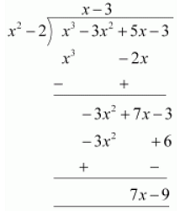Therefore, quotient = x – 3 and Remainder = 7x – 9

(ii)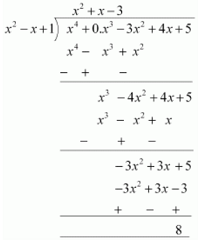Therefore, quotient = x² + x – 3 and Remainder = 8

(iii)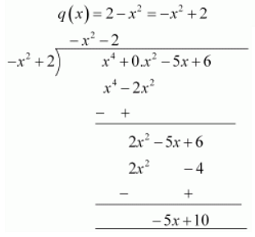Therefore, quotient = -x2 – 2 and, Remainder = −5x + 10

2. Check whether the first polynomial is a factor of the second polynomial by dividing the second polynomial by the first polynomial.

(i) t2 – 3, 2t4 + 3t2 – 9t -12

(ii) x2 + 3x +1, 3x4 + 5x3 – 7x2 + 2x + 2

(iii) x3 – 3x + 1, x5 – 4x3 + x2 + 3x +1

(i) t2 – 3, 2t4 + 3t2 – 9t -12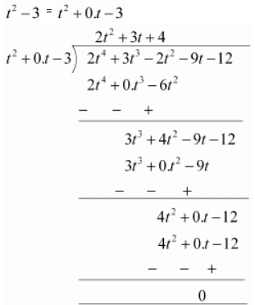Remainder = 0

Hence first polynomial is a factor of second polynomial.

(ii) x2 + 3x +1, 3x4 + 5x3 – 7x2 + 2x + 2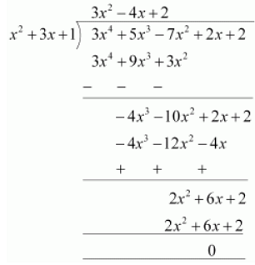Remainder = 0

Hence first polynomial is a factor of second polynomial.

(iii)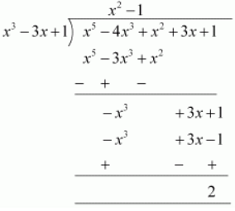Remainder ≠0

Hence first polynomial is not factor of second polynomial.

3. Obtain all other zeroes of ( 3×4 + 6×3 – 2×2 – 10x – 5 ) , if two of its zeroes are √5/3 and -√5/3.

Two zeroes of ( 3x4 + 6x– 2x2 – 10x – 5 ) are √5/3 and -√5/3 which means that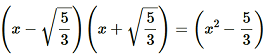is a factor of ( 3x4 + 6x– 2x2 – 10x – 5 ).

Therefore, we divide the given polynomial by x² – 5/3.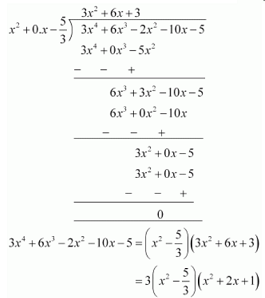we factorize x² + 2x + 1 = (x + 1)²

Therefore, its zero is given by x + 1 = 0

⇒ x = – 1

As it has the term (x + 1)²,therefore, there will be 2 zeros at x = – 1.

Hence,the zeroes of the given polynomial √5/3 and -√5/3  are −1 and −1.

4. On dividing ( x3 – 3x2 + x + 2 ) by a polynomial g(x), the quotient and remainder were (x-2) and (-2x+4) respectively. Find g(x).

Let p(x) = x3 – 3x2 + x + 2, q(x) = (x – 2) and r(x) = (–2x+4)

According to Polynomial Division Algorithm, we have

p(x) = g(x).q(x) + r(x)

⇒ x3 – 3x2 + x + 2 =g(x).(x−2)−2x+4

⇒x3 – 3x2 + x + 2 + 2x −4 =g(x).(x−2)

⇒x3 – 3x2 + 3x + 2=g(x).(x−2)

So, Dividing x3 – 3x2 + 3x + 2 by (x−2), we get

(x2 – x + 1)

Therefore, we haveg(x) = (x2 – x + 1)

5. Give examples of polynomials p(x), g(x), q(x) and r(x), which satisfy the division algorithm and

(i) deg p(x) = deg q(x)

(ii) deg q(x) = deg r(x)

(iii) deg r(x) = 0

Here let us represent, p(x) =dividend=the number to be divided

g(x)=Divisor=the number by which dividend is divided

q(x)=quotient

And r(x)=remainder

Now we have to give examples of polynomials such that the division algorithm

Dividend=Divisor × Quotient +Remainder

Is satisfies and the given conditions are also satisfied

(i)Let us assume the division of 2x + 4 by 2,

Then p(x) = 2x + 4

g(x) = 2

q(x) = x + 2

and r(x) = 0

On using the division algorithm

Dividend=Divisor × Quotient +Remainder

p(x)=g(x) × q(x) +r(x)

On putting the given values we get,

=> 2x + 4 = 2 x (x +2) + 0

On solving we get,

=> 2x + 4 = 2x + 4

Hence the division algorithm is satisfied.

And here the degree of p(x) =1 = degree of q(x)

Hence (i) condition is also satisfied.

(ii) Let us assume the division of x3 + x byx2

Then here,p(x) = x3+ x

g(x) = x2

q(x) = x

r(x) = x

It is clear that the degree of q(x) = 1 and

degree of r(x) = 1

on using the devision algorithm

=> Dividend=Divisor × Quotient +Remainder

=> p(x)=g(x) × q(x) +r(x)

On putting the given values we get,

=> x3 + x = (x2 * x) + x

on solving we get,

x3 + x = x 3 + x

Hence the division algorithm is satisfied.

and here the degree of r(x) = 1 = degree of q(x)

Hence (ii) condition is also satisfied.

(iii)Let us assume the division of x2 + 1 byx

Then here,p(x) = x2 + 1

g(x) = x

r(x) = 1

It is clear that the degree of r(x) = 0 and

on using the devision algorithm

=> Dividend=Divisor × Quotient +Remainder

=> p(x)=g(x) × q(x) +r(x)

On putting the given values we get,

=> x2 + 1 = (x * x)+ 1

x2 + 1 = x2 + 1

Hence,the division algorithm is satisfied.

And here the degree of r(x) = 0

Hence (iii) condition is also satisfied.

Here you can also assume any other polynomial for division but it is necessary that the chosen dividend and divisor be such that the conditions of the questions are satisfied.